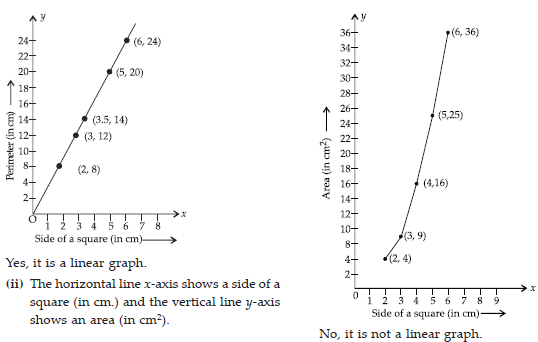# Introduction to Graphs (Mathematics) Class 8 - NCERT Questions

Q 1.

The following graph shows the temperature of a patient in a hospital, recorded every hour.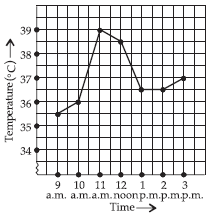(A) What was the patient’s temperature at 1 p.m.?
(B) When was the patient’s temperature 38.5° C?
(C) The patient’s temperature was the same two times during the period given. What were these two times ?
(D) What was the temperature at 1.30 p.m. ? How did you arrive at your answer?
(E) During which periods did the patient’s temperature showed an upward trend?

SOLUTION:

(A) At 1 p.m. patient’s temperature was 36.5°C.
(B) At 12 noon the patient’s temperature was 38.5°C.
(C) The patient’s temperature was 36.5°C at 1 p.m. and 2 p.m.
(D) The patient’s temperature was 36.5°C at 1.30 pm (because the temperature of the patient was constant from 1 p.m. to 2 p.m.). The point between 1 p.m. and 2 p.m. on the x-axis is equidistant from the two points showing 1 p.m. and 2 p.m., so it will represent 1 : 30 p.m. Similarly, the point on the y-axis, between 36°C and 37°C will represent 36.5°C.
(E) 9 a.m. to 10 a.m., 10 a.m. to 11 a.m. and 2 p.m. to 3 p.m.

Q 2.

The following line graph shows the yearly sales figures for a manufacturing company.
(A) What were the sales in
(i) 2002 (ii) 2006?
(B) What were the sales in
(i) 2003 (ii) 2005?
(C) Compute the difference between the sales in 2002 and 2006.
(D) In which year was there the greatest difference between the sales as compared to its previous year?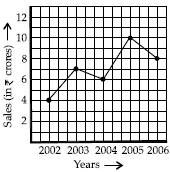SOLUTION:

(A) (i) In 2002 company sales were ` 4 crores,
(ii) In 2006 company sales were ` 8 crores. (B) (i) The sales of a manufacturing company in 2003 were ` 7 crores.
(ii) The sales of a manufacturing company in 2005 were ` 10 crores.
(C) The difference between the sales in 2002 and 2006.
= Sales in 2006 – Sales in 2002
= ` 8 crores – ` 4 crores = ` 4 crores.
(D) In year 2005, the difference between the sales was greatest as compared to its previous year.

Q 3.

For an experiment in Botany, two different plants, plant A and plant B were grown under similar laboratory conditions. Their heights were measured at the end of each week for 3 weeks. The results are shown by the following graph.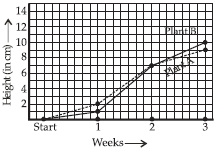(A) How high was Plant A after
(i) 2 weeks, (ii) 3 weeks?
(B) How high was Plant B after
(i) 2 weeks, (ii) 3 weeks?
(C) How much did Plant A grow during the 3rd week?
(D) How much did Plant B grow from the end of the 2nd week to the end of the 3rd week?
(E) During which week did Plant A grow most?
(f) During which week did Plant B grow least?
(g) Were the two plants of the same height during any week shown here? Specify.

SOLUTION:

(A) (i) After 2 weeks the height of plant A was 7 cm.
(ii) After 3 weeks the height of plant A was 9 cm.
(B) (i) After 2 weeks the height of plant B was 7 cm.
(ii) After 3 weeks the height of plant B was 10 cm. (C) During the 3rd week plant A grew (9 – 7) cm = 2 cm.
(D) The plant B grow from the end of 2nd week to the end of 3rd week = 10 cm – 7 cm = 3 cm
(E) The plant A grew most during second week.
(f) The plant B grew least during first week.
(g) Yes, at the end of second week.

Q 4.

The following graph shows the temperature forecast and the actual temperature for each day of a week.
(A) On which days was the forecast temperature the same as the actual temperature?
(B) What was the maximum forecast temperature during the week?
(C) What was the minimum actual temperature during the week?
(D) On which day did the actual temperature differ the most from the forecast temperature?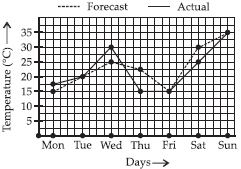SOLUTION:

(A) The forecast temperature is the same as actual temperature on :
Tuesday, Friday and Sunday.
(B) The maximum forecast temperature during the week was 35°C.
(C) The minimum actual temperature during the week was 15°C.
(D) On Thursday, the actual temperature was differ the most from the forecast temperature.

Q 5.

Use the tables below to draw linear graphs.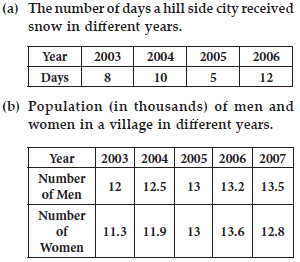SOLUTION:

(A) By using a given information we draw a line graph, where the horizontal line i.e. x-axis shows the year and the vertical line i.e. y-axis shows the number of days.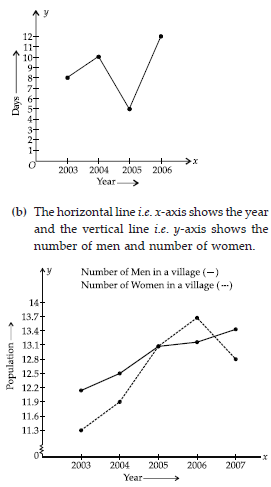Q 6.

A courier-person cycles from a town to a neighbouring suburban area to deliver a parcel to a merchant. His distance from the town at different times is shown by the following graph.
(A) What is the scale taken for the time axis?
(B) How much time did the person take for the travel?
(C) How far is the place of the merchant from the town?
(D) Did the person stop on his way? Explain.
(E) During which period did he ride fastest?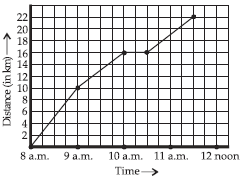SOLUTION: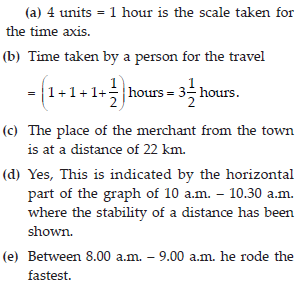Q 7.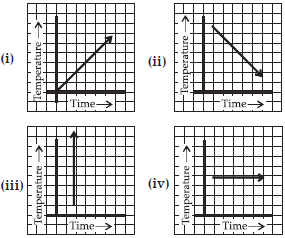SOLUTION:

Figure (iii) is not a possible time - temperature graph. Since, at the same time, temperature can’t be different.

Q 8.

Plot the following points on a graph sheet. Verify if they lie on a line
(A) A(4, 0), B(4, 2), C(4, 6), D(4, 2.5)
(B) P(1,1), Q(2, 2), R(3, 3), S(4, 4)
(C) K(2, 3), L(5, 3), M(5, 5), N(2, 5).

SOLUTION: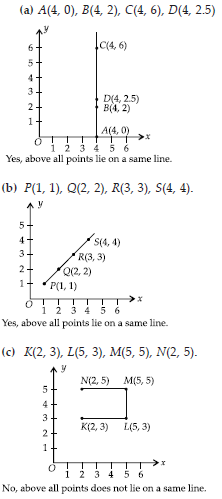Q 9.

Draw the line passing through (2, 3) and (3, 2). Find the coordinates of the points at which this line meets the x-axis and y-axis.

SOLUTION:

After drawing a graph the line passing through (2, 3) and (3, 2), then the co-ordinates of the points which meets the x-axis and y-axis is at (5, 0) and (0, 5) respectively.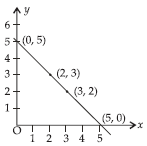Q 10.

Write the coordinates of the vertices of each of these adjoining figures.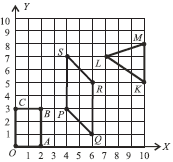SOLUTION:

After observing a graph we find that O(0, 0), A(2, 0), B(2, 3), C(0, 3), P(4, 3), Q(6, 1), R(6, 5), S(4, 7), L(7, 7), M(10, 8), K(10, 5).

Q 11.

State whether True or False. Correct that are false.
(i) A point whose x-coordinate is zero and y-coordinate is non-zero will lie on the y-axis.
(ii) A point whose y-coordinate is zero and x-coordinate is 5 will lie on y-axis.
(iii) The coordinates of the origin are (0, 0).

SOLUTION:

(i) True (ii) False, because it will lie on x-axis. (iii) True.

Q 12.

Draw the graphs for the following tables of values, with suitable scales on the axes.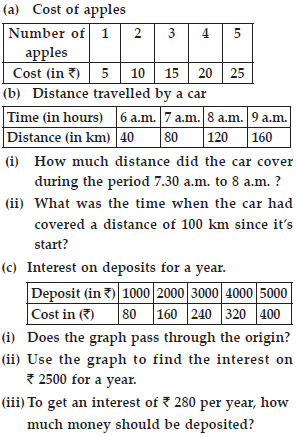SOLUTION: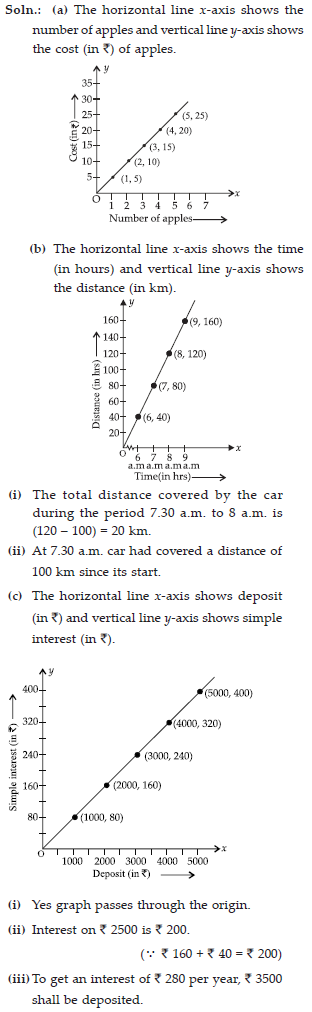Q 13.

Draw a graph for the followingSOLUTION:

(i) The horizontal line x-axis shows a side of square (in cm) and the vertical line y-axis shows a perimeter (in cm).